# NCERT Exemplar Class 11 Physics Solutions for Chapter 2 - Units And Measurements

NCERT Exemplar Class 11 physics Chapter 2 Units and Measurements are presented here for the benefit of the student in their CBSE Class 11 examination and competitive examinations. Chapter 2 Units and Measurements is basic to all your future endeavours so it is very important to understand and memorise every concept in this chapter.

NCERT exemplar Class 11 physics Chapter 2 pdf will help you get profound with Units and measurement. It consists of questions from NCERT exemplar book along with extra questions prepared by subject experts and BYJU’S. NCERT exemplar class 11 physics Chapter 2 also comprise important questions from previous year question papers and sample papers.

The comparison of a standard unit of any physical quantity with a standard unit is defined as the measurement. The laws of physics that are described in terms of quantities whose measurement is necessary are titled as physical quantities. A standard unit should be universally accepted and easily reproducible as a definite amount.

Some of the fundamental units

1. Mass
2. Time
3. Temperature
4. Length
5. Electric current

## Download the PDF of NCERT Exemplar Class 11 Physics Chapter 2 Units and Measurements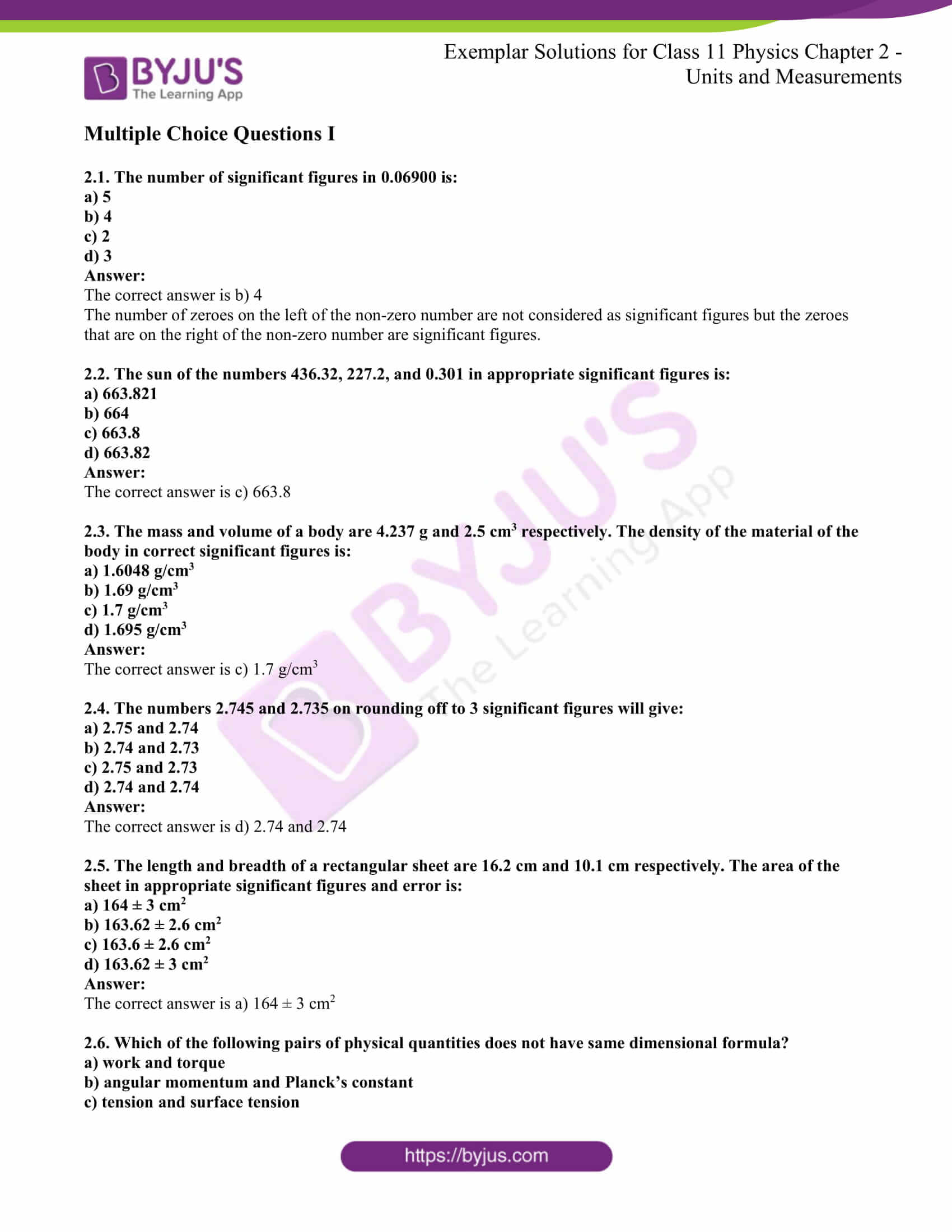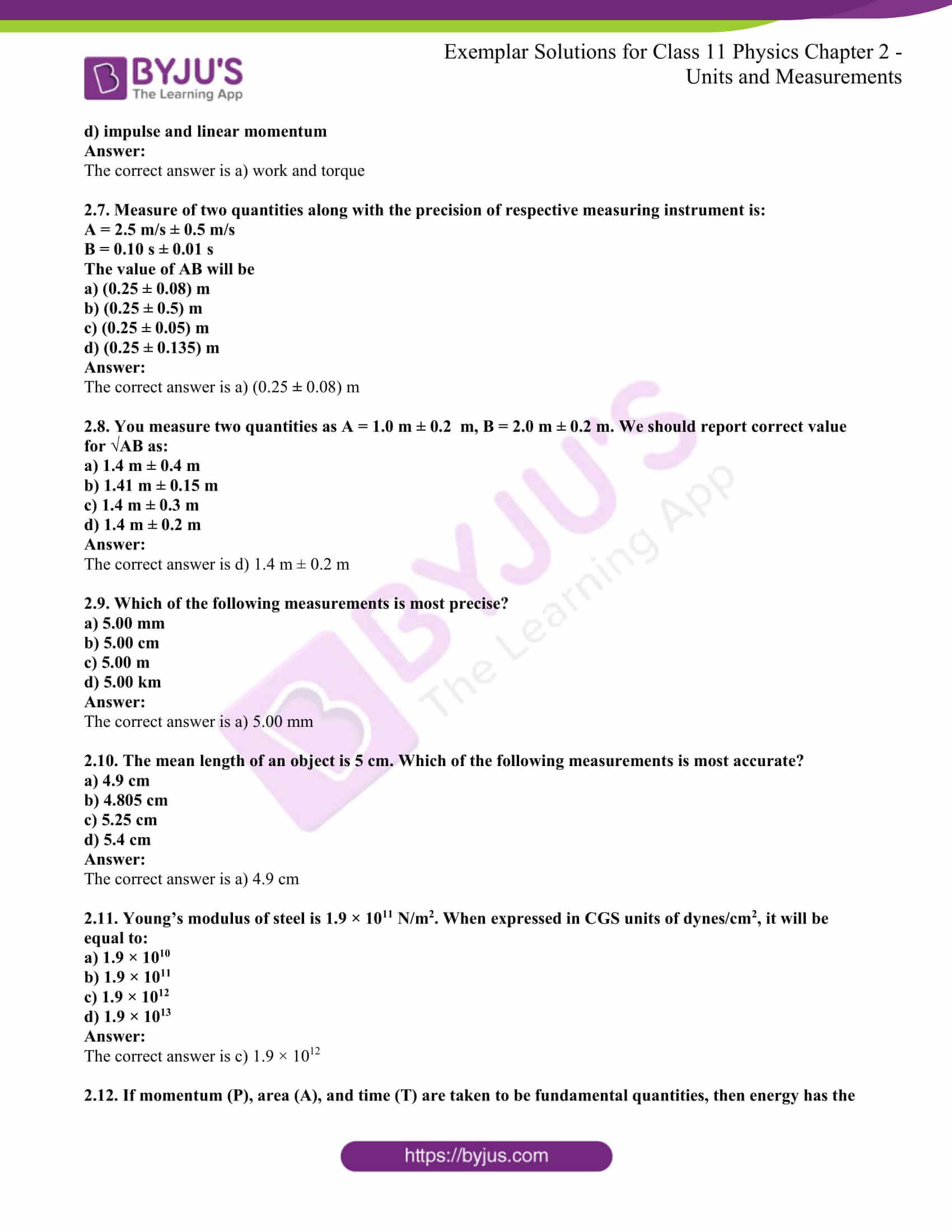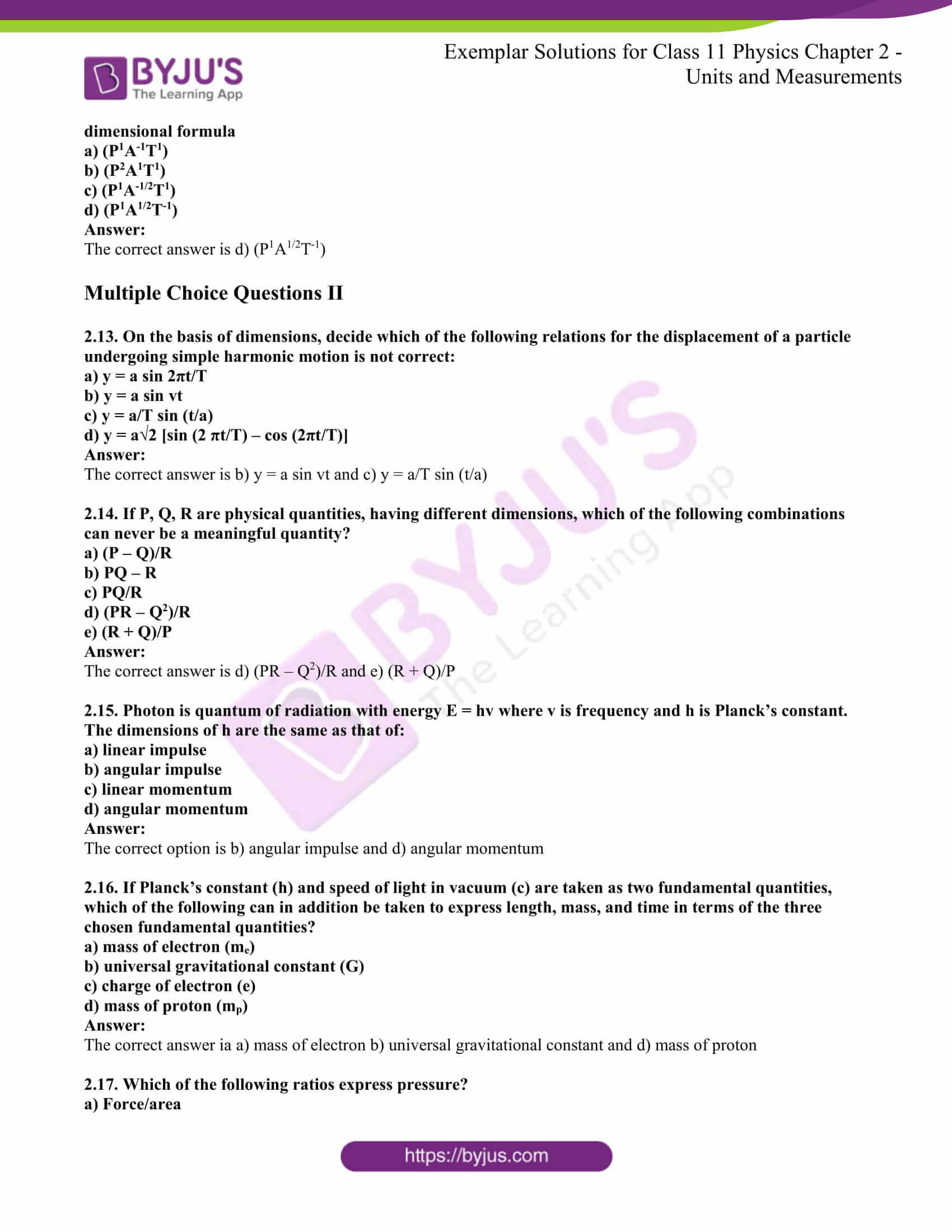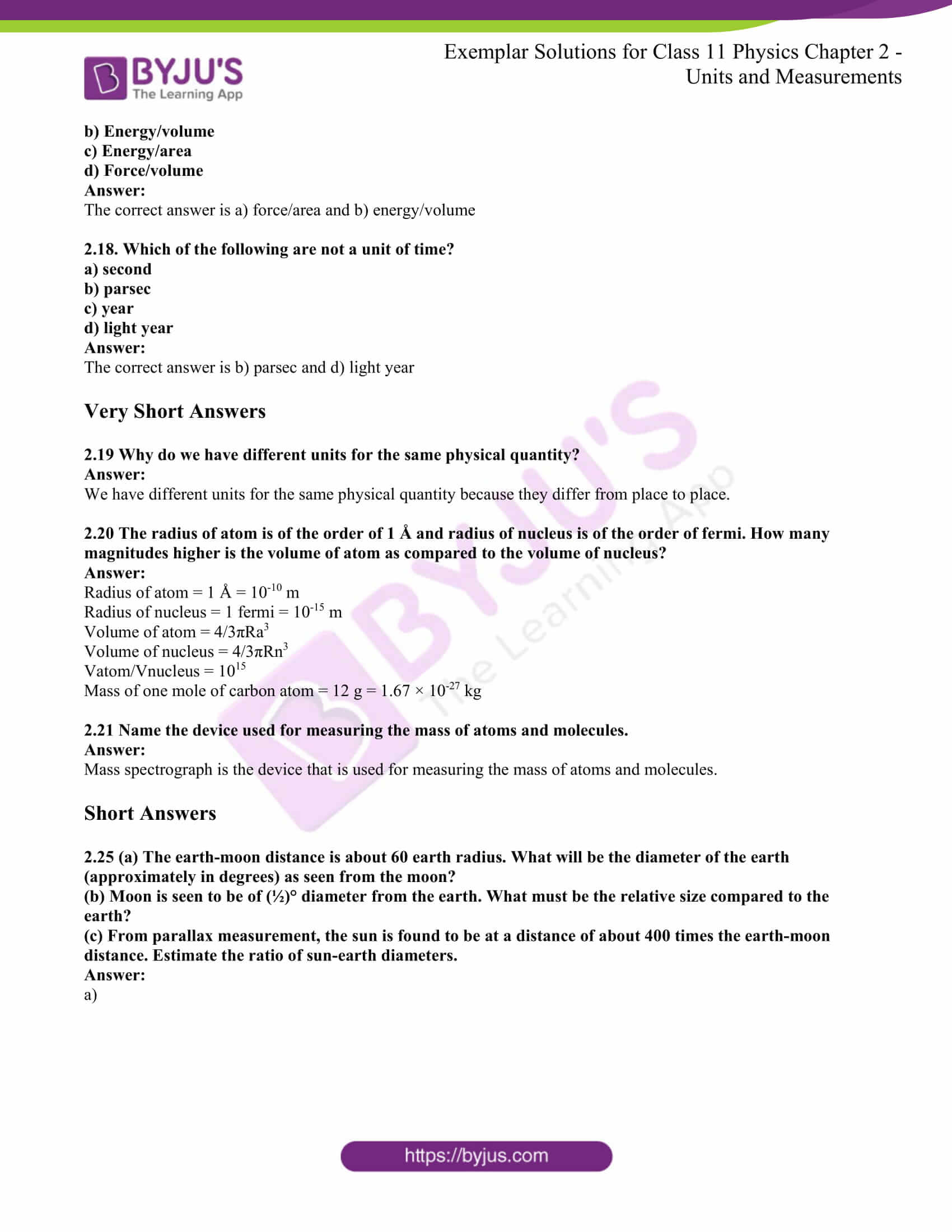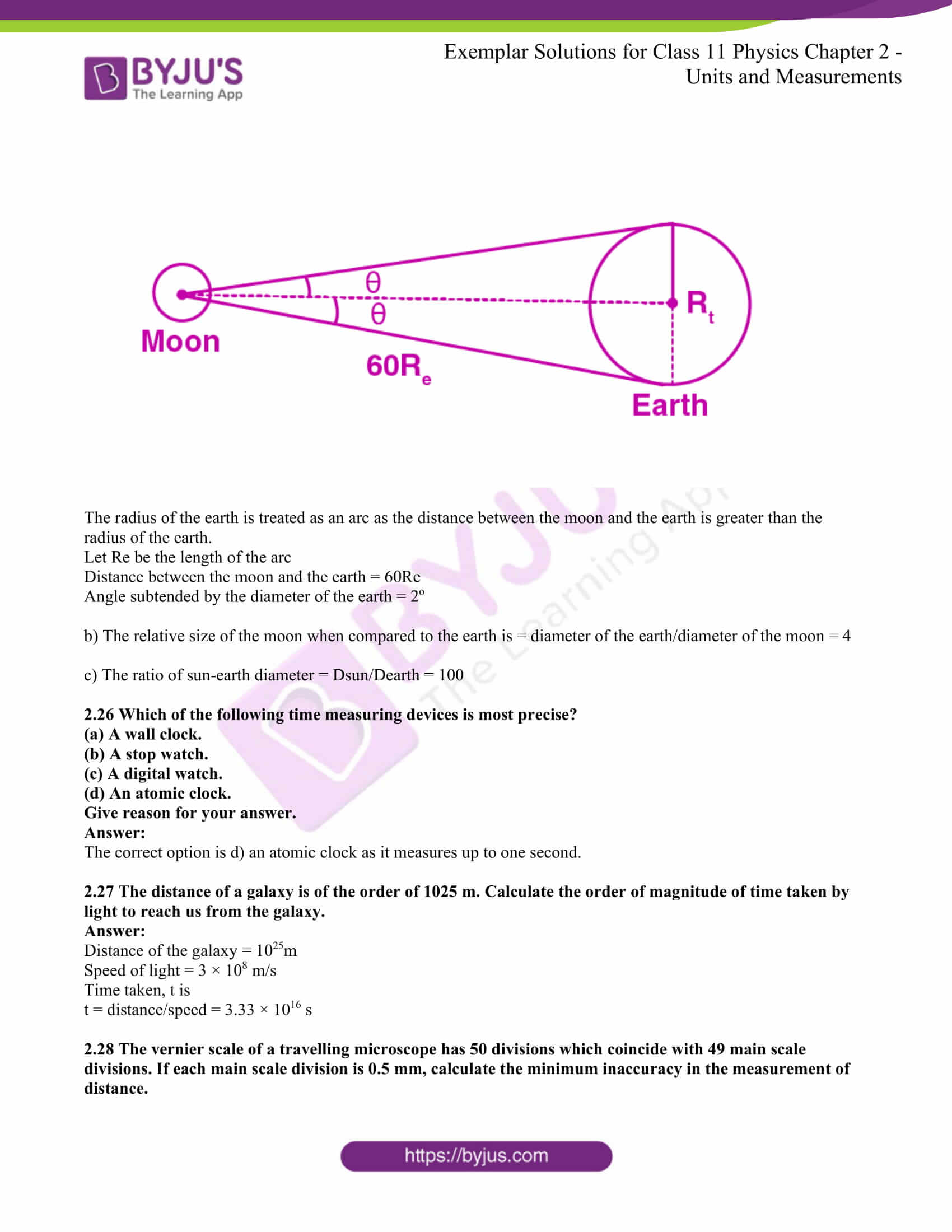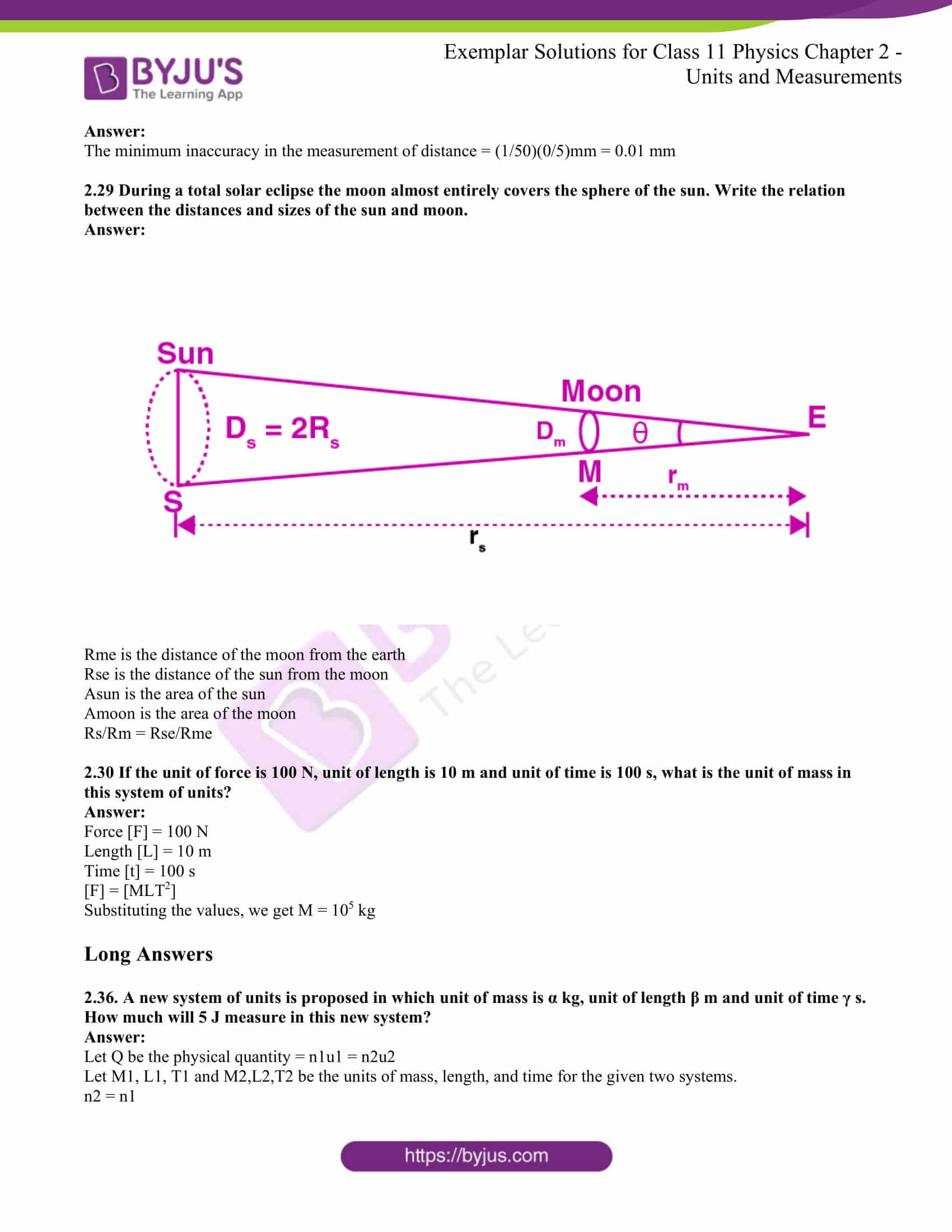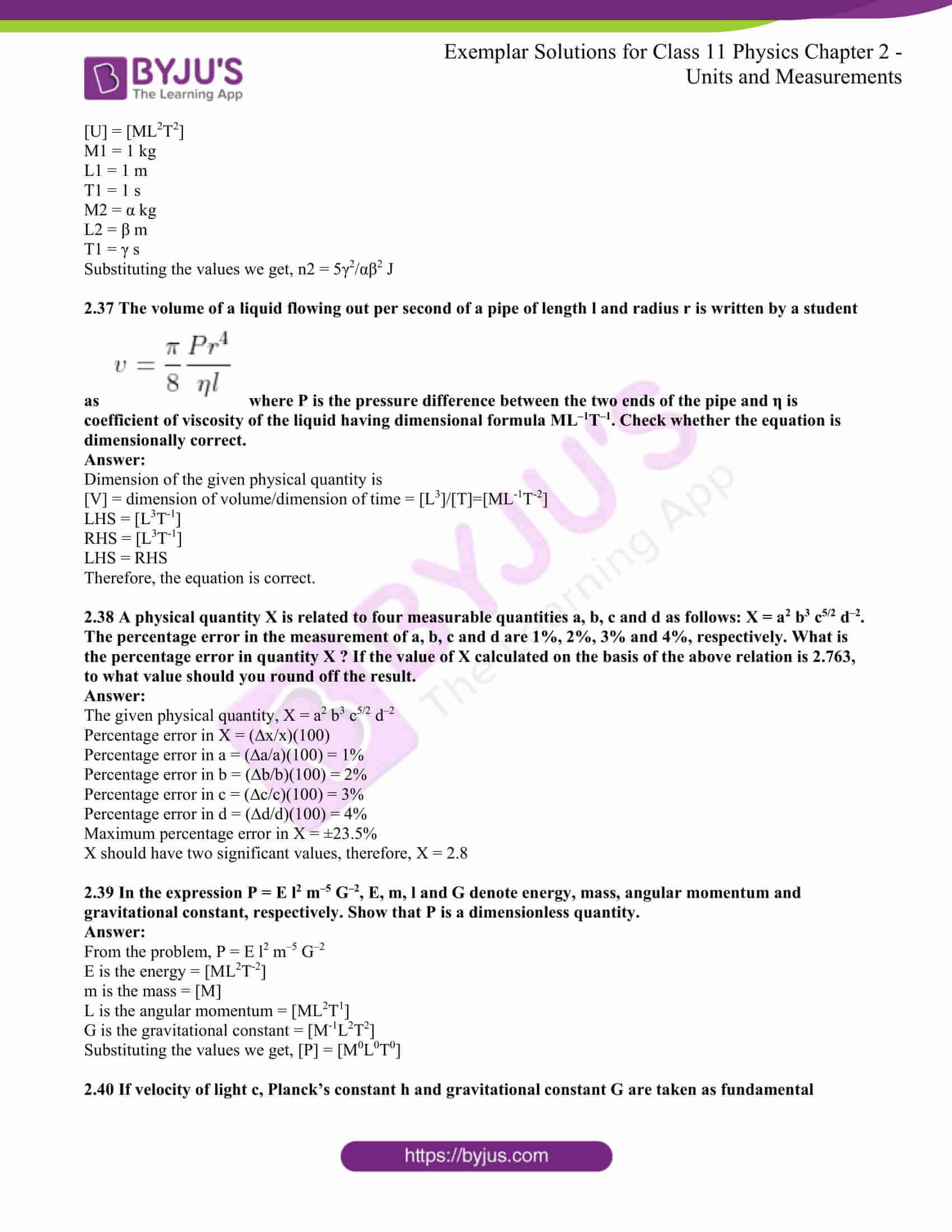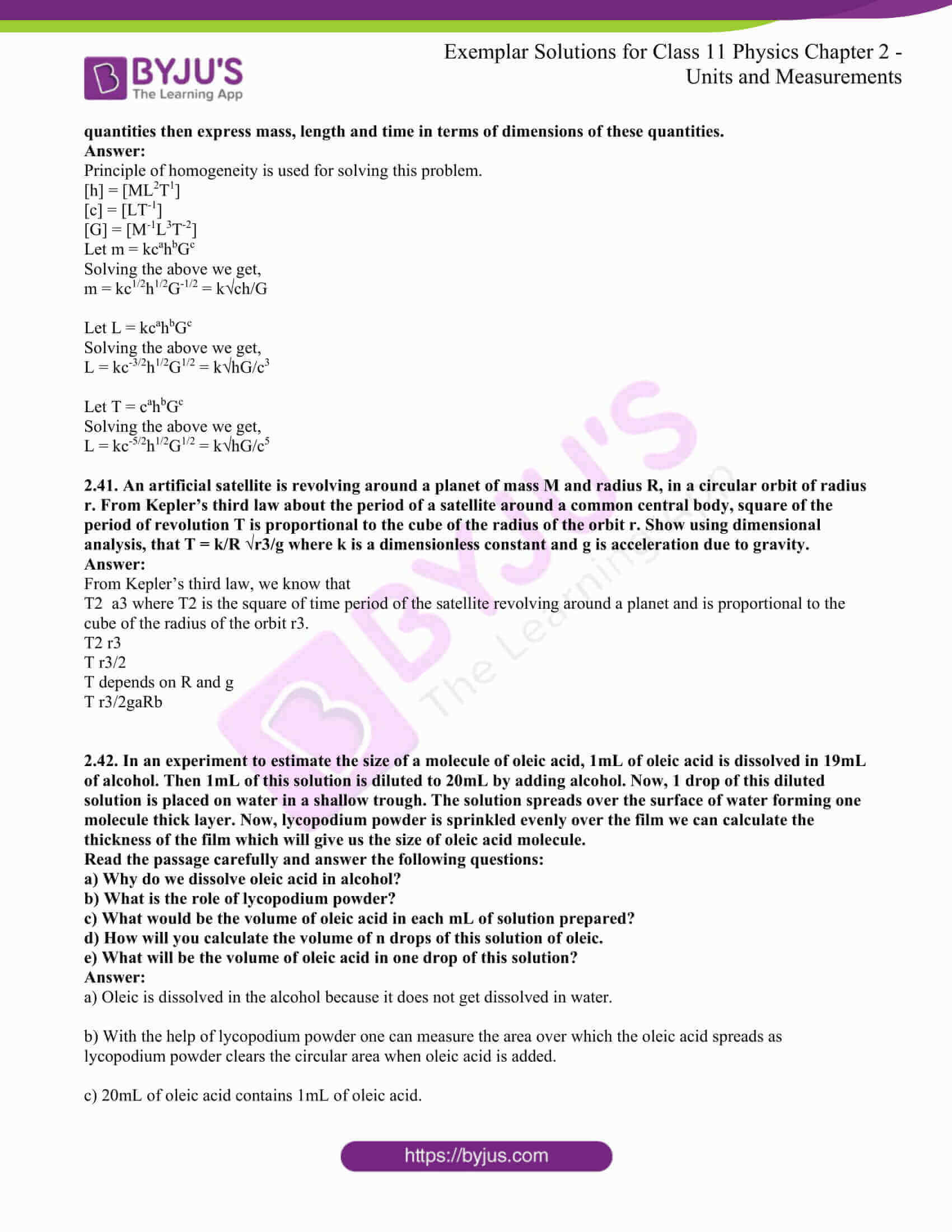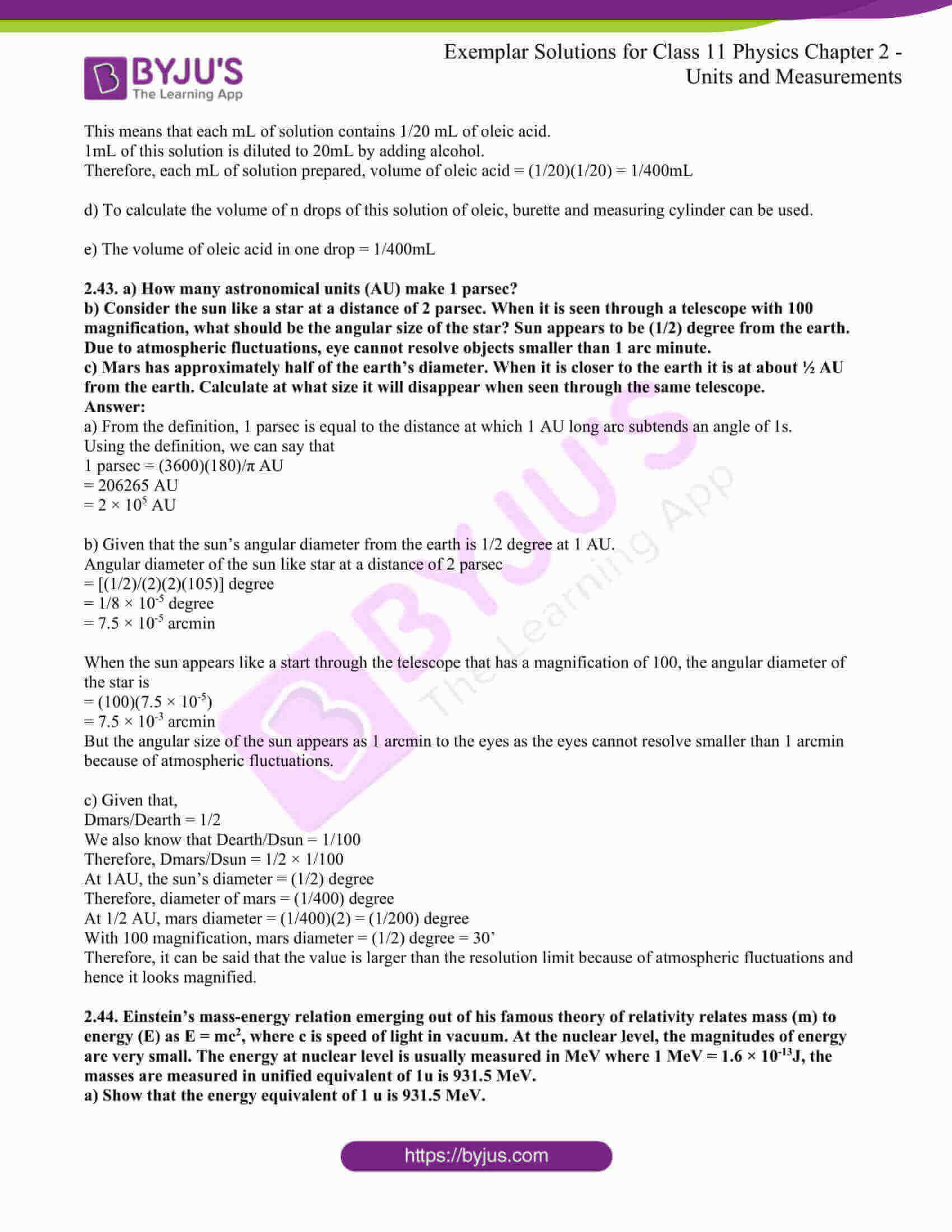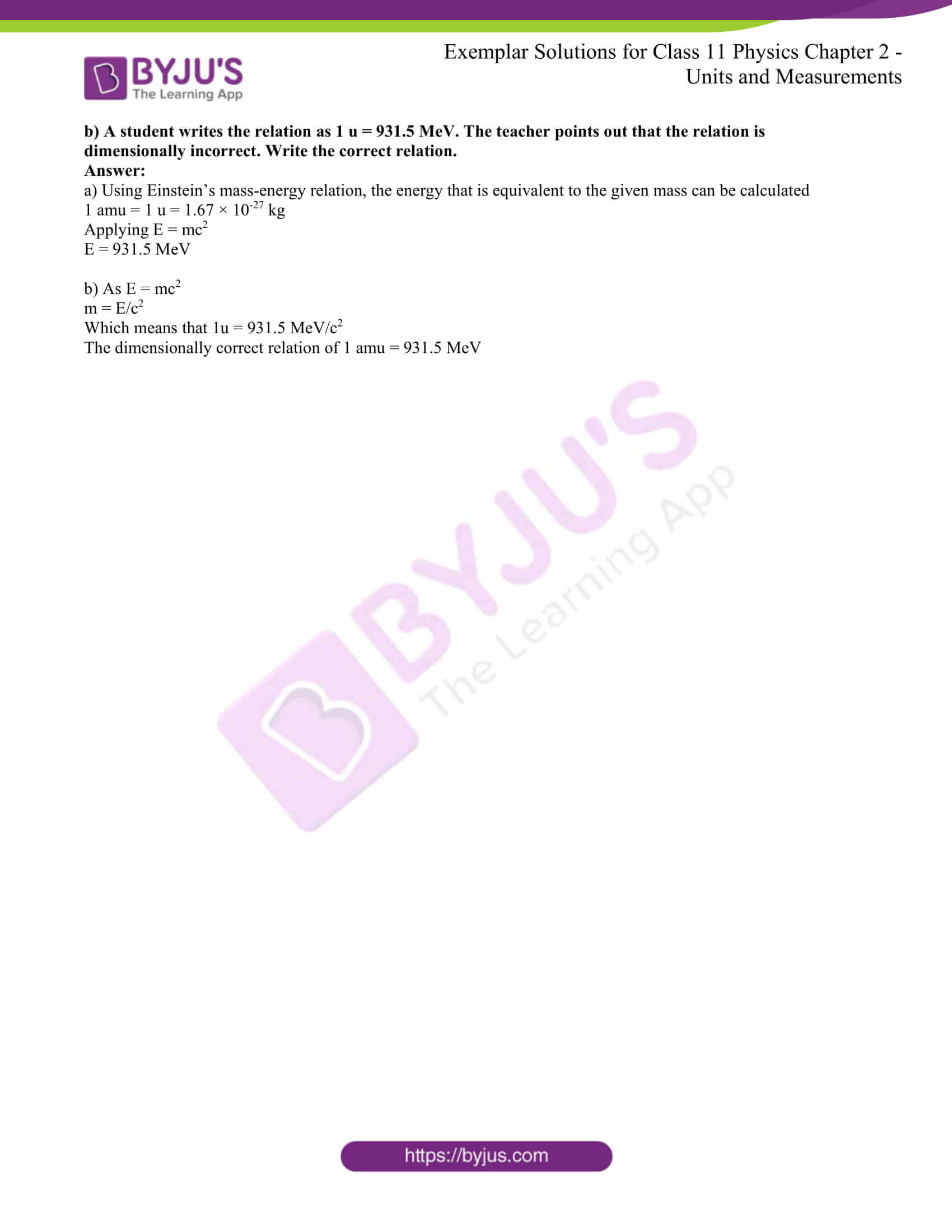### Multiple Choice Questions I

2.1. The number of significant figures in 0.06900 is:

a) 5

b) 4

c) 2

d) 3

The correct answer is b) 4

The number of zeroes on the left of the non-zero number is not considered as significant figures but the zeroes that are on the right of the non-zero number are significant figures.

2.2. The sun of the numbers 436.32, 227.2, and 0.301 inappropriate significant figures is:

a) 663.821

b) 664

c) 663.8

d) 663.82

The correct answer is c) 663.8

2.3. The mass and volume of a body are 4.237 g and 2.5 cm3 respectively. The density of the material of the body in correct significant figures is:

a) 1.6048 g/cm3

b) 1.69 g/cm3

c) 1.7 g/cm3

d) 1.695 g/cm3

The correct answer is c) 1.7 g/cm3

2.4. The numbers 2.745 and 2.735 on rounding off to 3 significant figures will give:

a) 2.75 and 2.74

b) 2.74 and 2.73

c) 2.75 and 2.73

d) 2.74 and 2.74

The correct answer is d) 2.74 and 2.74

2.5. The length and breadth of a rectangular sheet are 16.2 cm and 10.1 cm respectively. The area of the sheet inappropriate significant figures and error is:

a) 164 ± 3 cm2

b) 163.62 ± 2.6 cm2

c) 163.6 ± 2.6 cm2

d) 163.62 ± 3 cm2

The correct answer is a) 164 ± 3 cm2

2.6. Which of the following pairs of physical quantities does not have the same dimensional formula?

a) work and torque

b) angular momentum and Planck’s constant

c) tension and surface tension

d) impulse and linear momentum

The correct answer is a) work and torque

2.7. Measure of two quantities along with the precision of the respective measuring instrument is:

A = 2.5 m/s ± 0.5 m/s

B = 0.10 s ± 0.01 s

The value of AB will be

a) (0.25 ± 0.08) m

b) (0.25 ± 0.5) m

c) (0.25 ± 0.05) m

d) (0.25 ± 0.135) m

The correct answer is a) (0.25 ± 0.08) m

2.8. You measure two quantities as A = 1.0 m ± 0.2 m, B = 2.0 m ± 0.2 m. We should report the correct value for √AB as:

a) 1.4 m ± 0.4 m

b) 1.41 m ± 0.15 m

c) 1.4 m ± 0.3 m

d) 1.4 m ± 0.2 m

The correct answer is d) 1.4 m ± 0.2 m

2.9. Which of the following measurements is most precise?

a) 5.00 mm

b) 5.00 cm

c) 5.00 m

d) 5.00 km

The correct answer is a) 5.00 mm

2.10. The mean length of an object is 5 cm. Which of the following measurements is most accurate?

a) 4.9 cm

b) 4.805 cm

c) 5.25 cm

d) 5.4 cm

The correct answer is a) 4.9 cm

2.11. Young’s modulus of steel is 1.9 × 1011 N/m2. When expressed in CGS units of dynes/cm2, it will be equal to:

a) 1.9 × 1010

b) 1.9 × 1011

c) 1.9 × 1012

d) 1.9 × 1013

The correct answer is c) 1.9 × 1012

2.12. If momentum (P), area (A), and time (T) are taken to be fundamental quantities, then energy has the dimensional formula

a) (P1A-1T1)

b) (P2A1T1)

c) (P1A-1/2T1)

d) (P1A1/2T-1)

The correct answer is d) (P1A1/2T-1)

### Multiple Choice Questions II

2.13. On the basis of dimensions, decide which of the following relations for the displacement of a particle undergoing simple harmonic motion is not correct:

a) y = a sin 2πt/T

b) y = a sin vt

c) y = a/T sin (t/a)

d) y = a√2 [sin (2 πt/T) – cos (2πt/T)]

The correct answer is b) y = a sin vt and c) y = a/T sin (t/a)

2.14. If P, Q, R are physical quantities, having different dimensions, which of the following combinations can never be a meaningful quantity?

a) (P – Q)/R

b) PQ – R

c) PQ/R

d) (PR – Q2)/R

e) (R + Q)/P

The correct answer is d) (PR – Q2)/R and e) (R + Q)/P

2.15. Photon is quantum of radiation with energy E = hv where v is frequency and h is Planck’s constant. The dimensions of h are the same as that of:

a) linear impulse

b) angular impulse

c) linear momentum

d) angular momentum

The correct option is b) angular impulse and d) angular momentum

2.16. If Planck’s constant (h) and speed of light in vacuum (c) are taken as two fundamental quantities, which of the following can, also, be taken to express length, mass, and time in terms of the three chosen fundamental quantities?

a) mass of the electron (me)

b) universal gravitational constant (G)

c) charge of the electron (e)

d) mass of proton (mp)

The correct answer is a) mass of electron b) universal gravitational constant and d) mass of proton

2.17. Which of the following ratios express pressure?

a) Force/area

b) Energy/volume

c) Energy/area

d) Force/volume

The correct answer is a) force/area and b) energy/volume

2.18. Which of the following are not a unit of time?

a) second

b) parsec

c) year

d) light year

The correct answer is b) parsec and d) light year

2.19 Why do we have different units for the same physical quantity?

We have different units for the same physical quantity because they differ from place to place.

2.20 The radius of an atom is of the order of 1 Å and radius of nucleus is of the order of fermi. How many magnitudes higher is the volume of the atom as compared to the volume of the nucleus?

Radius of atom = 1 Å = 10-10 m

Radius of nucleus = 1 fermi = 10-15 m

Volume of atom = 4/3πRa3

Volume of nucleus = 4/3πRn3

Vatom/Vnucleus = 1015

Mass of one mole of carbon atom = 12 g = 1.67 × 10-27 kg

2.21 Name the device used for measuring the mass of atoms and molecules.

Mass spectrograph is the device that is used for measuring the mass of atoms and molecules.

2.25 (a) The earth-moon distance is about 60 earth radius. What will be the diameter of the earth (approximately in degrees) as seen from the moon?

(b) Moon is seen to be of (½)° diameter from the earth. What must be the relative size compared to the earth?

(c) From parallax measurement, the sun is found to be at a distance of about 400 times the earth-moon distance. Estimate the ratio of sun-earth diameters.

a)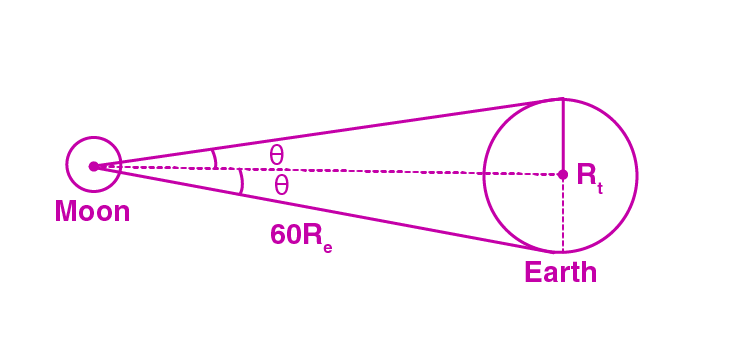The radius of the earth is treated as an arc as the distance between the moon and the earth is greater than the radius of the earth.

Let Re be the length of the arc

Distance between the moon and the earth = 60Re

Angle subtended by the diameter of the earth = 2o

b) The relative size of the moon, when compared to the earth, is = diameter of the earth/diameter of the moon = 4

c) The ratio of sun-earth diameter = Dsun/Dearth = 100

2.26 Which of the following time measuring devices is most precise?

(a) A wall clock.

(b) A stopwatch.

(c) A digital watch.

(d) An atomic clock.

The correct option is d) an atomic clock as it measures up to one second.

2.27 The distance of a galaxy is of the order of 1025 m. Calculate the order of magnitude of time taken by light to reach us from the galaxy.

Distance of the galaxy = 1025m

Speed of light = 3 × 108 m/s

Time taken, t is

t = distance/speed = 3.33 × 1016 s

2.28 The vernier scale of a travelling microscope has 50 divisions which coincide with 49 main scale divisions. If each main scale division is 0.5 mm, calculate the minimum inaccuracy in the measurement of distance.

The minimum inaccuracy in the measurement of distance = (1/50)(0/5)mm = 0.01 mm

2.29 During a total solar eclipse-the moon almost entirely covers the sphere of the sun. Write the relation between the distances and sizes of the sun and moon.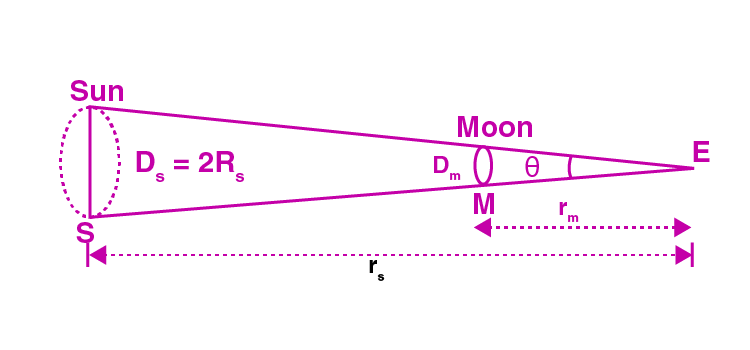Rme is the distance of the moon from the earth

Rse is the distance of the sun from the moon

Asun is the area of the sun

Amoon is the area of the moon

Rs/Rm = Rse/Rme

2.30 If the unit of force is 100 N, unit of length is 10 m and unit of time is 100 s, what is the unit of mass in this system of units?

Force [F] = 100 N

Length [L] = 10 m

Time [t] = 100 s

[F] = [MLT2]

Substituting the values, we get M = 105 kg

2.36. A new system of units is proposed in which unit of mass is α kg, unit of length β m and unit of time γ s. How much will 5 J measure in this new system?

Let Q be the physical quantity = n1u1 = n2u2

Let M1, L1, T1 and M2,L2,T2 be the units of mass, length, and time for the given two systems.

n2 = n1

[U] = [ML2T2]

M1 = 1 kg

L1 = 1 m

T1 = 1 s

M2 = α kg

L2 = β m

T1 = γ s

Substituting the values we get, n2 = 5γ2/αβ2 J

2.37 The volume of a liquid flowing out per second of a pipe of length l and radius r is written by a student as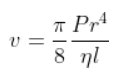where P is the pressure difference between the two ends of the pipe and η is coefficient of viscosity of the liquid having dimensional formula ML–1T–1. Check whether the equation is dimensionally correct.

Dimension of the given physical quantity is

[V] = dimension of volume/dimension of time = [L3]/[T]=[ML-1T-2]

LHS = [L3T-1]

RHS = [L3T-1]

LHS = RHS

Therefore, the equation is correct.

2.38 A physical quantity X is related to four measurable quantities a, b, c and d as follows: X = a2 b3 c5/2 d–2. The percentage error in the measurement of a, b, c and d are 1%, 2%, 3% and 4%, respectively. What is the percentage error in quantity X? If the value of X calculated on the basis of the above relation is 2.763, to what value should you round off the result.

The given physical quantity, X = a2 b3 c5/2 d–2

Percentage error in X = (∆x/x)(100)

Percentage error in a = (∆a/a)(100) = 1%

Percentage error in b = (∆b/b)(100) = 2%

Percentage error in c = (∆c/c)(100) = 3%

Percentage error in d = (∆d/d)(100) = 4%

Maximum percentage error in X = ±23.5%

X should have two significant values, therefore, X = 2.8

2.39 In the expression P = E l2 m–5 G–2, E, m, l and G denote energy, mass, angular momentum and gravitational constant, respectively. Show that P is a dimensionless quantity.

From the problem, P = E l2 m–5 G–2

E is the energy = [ML2T-2]

m is the mass = [M]

L is the angular momentum = [ML2T1]

G is the gravitational constant = [M-1L2T2]

Substituting the values we get, [P] = [M0L0T0]

2.40 If the velocity of light c, Planck’s constant h and gravitational constant G are taken as fundamental quantities then express mass, length and time in terms of dimensions of these quantities.

Principle of homogeneity is used for solving this problem.

[h] = [ML2T1] [c] = [LT-1] [G] = [M-1L3T-2]

Let m = kcahbGc

Solving the above we get,

m = kc1/2h1/2G-1/2 = k√ch/G

Let L = kcahbGc

Solving the above we get,

L = kc-3/2h1/2G1/2 = k√hG/c3

Let T = cahbGc

Solving the above we get,

L = kc-5/2h1/2G1/2 = k√hG/c5

2.41. An artificial satellite is revolving around a planet of mass M and radius R, in a circular orbit of radius r. From Kepler’s third law about the period of a satellite around a common central body, square of the period of revolution T is proportional to the cube of the radius of the orbit r. Show using dimensional analysis, that T = k/R √r3/g where k is a dimensionless constant and g is acceleration due to gravity.

From Kepler’s third law, we know that

T2 a3 where T2 is the square of time period of the satellite revolving around a planet and is proportional to the cube of the radius of the orbit r3.

T2 r3

T r3/2

T depends on R and g

T r3/2gaRb

2.42. In an experiment to estimate the size of a molecule of oleic acid, 1mL of oleic acid is dissolved in 19mL of alcohol. Then 1mL of this solution is diluted to 20mL by adding alcohol. Now, 1 drop of this diluted solution is placed on water in a shallow trough. The solution spreads over the surface of water forming one molecule thick layer. Now, lycopodium powder is sprinkled evenly over the film we can calculate the thickness of the film which will give us the size of oleic acid molecule.

a) Why do we dissolve oleic acid in alcohol?

b) What is the role of lycopodium powder?

c) What would be the volume of oleic acid in each mL of solution prepared?

d) How will you calculate the volume of n drops of this solution of oleic.

e) What will be the volume of oleic acid in one drop of this solution?

a) Oleic is dissolved in the alcohol because it does not get dissolved in water.

b) With the help of lycopodium powder one can measure the area over which the oleic acid spreads as lycopodium powder clears the circular area when oleic acid is added.

c) 20mL of oleic acid contains 1mL of oleic acid.

This means that each mL of solution contains 1/20 mL of oleic acid.

1mL of this solution is diluted to 20mL by adding alcohol.

Therefore, each mL of solution prepared, volume of oleic acid = (1/20)(1/20) = 1/400mL

d) To calculate the volume of n drops of this solution of oleic, burette and measuring cylinder can be used.

e) The volume of oleic acid in one drop = 1/400mL

2.43. a) How many astronomical units (AU) make 1 parsec?

b) Consider the sun like a star at a distance of 2 parsec. When it is seen through a telescope with 100 magnification, what should be the angular size of the star? Sun appears to be (1/2) degree from the earth. Due to atmospheric fluctuations, eye cannot resolve objects smaller than 1 arc minute.

c) Mars has approximately half of the earth’s diameter. When it is closer to the earth it is at about ½ AU from the earth. Calculate at what size it will disappear when seen through the same telescope.

a) From the definition, 1 parsec is equal to the distance at which 1 AU long arc subtends an angle of 1s.

Using the definition, we can say that

1 parsec = (3600)(180)/π AU

= 206265 AU

= 2 × 105 AU

b) Given that the sun’s angular diameter from the earth is 1/2 degree at 1 AU.

Angular diameter of the sun like star at a distance of 2 parsec

= [(1/2)/(2)(2)(105)] degree

= 1/8 × 10-5 degree

= 7.5 × 10-5 arcmin

When the sun appears like a start through the telescope that has a magnification of 100, the angular diameter of the star is

= (100)(7.5 × 10-5)

= 7.5 × 10-3 arcmin

But the angular size of the sun appears as 1 arcmin to the eyes as the eyes cannot resolve smaller than 1 arcmin because of atmospheric fluctuations.

c) Given that,

Dmars/Dearth = 1/2

We also know that Dearth/Dsun = 1/100

Therefore, Dmars/Dsun = 1/2 × 1/100

At 1AU, the sun’s diameter = (1/2) degree

Therefore, diameter of mars = (1/400) degree

At 1/2 AU, mars diameter = (1/400)(2) = (1/200) degree

With 100 magnification, mars diameter = (1/2) degree = 30’

Therefore, it can be said that the value is larger than the resolution limit because of atmospheric fluctuations and hence it looks magnified.

2.44. Einstein’s mass-energy relation emerging out of his famous theory of relativity relates mass (m) to energy (E) as E = mc2, where c is the speed of light in vacuum. At the nuclear level, the magnitudes of energy are very small. The energy at nuclear level is usually measured in MeV where 1 MeV = 1.6 × 10-13J, the masses are measured in unified equivalent of 1u is 931.5 MeV.

a) Show that the energy equivalent of 1 u is 931.5 MeV.

b) A student writes the relation as 1 u = 931.5 MeV. The teacher points out that the relation is dimensionally incorrect. Write the correct relation.

a) Using Einstein’s mass-energy relation, the energy that is equivalent to the given mass can be calculated

1 amu = 1 u = 1.67 × 10-27 kg

Applying E = mc2

E = 931.5 MeV

b) As E = mc2

m = E/c2

Which means that 1u = 931.5 MeV/c2

The dimensionally correct relation of 1 amu = 931.5 MeV

### Class 11 Chapter 2 Physics Exemplar Concepts for Units and Measurement

 Section Number Topic 1 The International System Of Units 2. Measurement Of Length 3 Measurement Of Mass 4 Measurement Of Time 5 Accuracy, Precision Of Instruments And Errors In Measurement 6 Significant Figures 7 Dimensions Of Physical Quantities 8 Dimensional Formulae And Dimensional Equations 9 Dimensional Analysis And Its Applications

### Why Opt for BYJU’S?

BYJU’S motto is to create top-quality learning content for the students. With the focus to boost student’s learning capabilities, NCERT Exemplar provided here are framed by our experts with over a decade of experience. BYJU’S NCERT Solutions for Class 11 Physics Chapter 11 will help students practice and revise the different topics right from their homes.